# Top 30 Apple coding interview questions (with solutions)

Mar 26, 2021 - 20 min read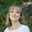Amanda FawcettWorking at Apple is a dream for many developers, but preparing for coding interviews is no easy task. To make your life easier, we’ve compiled the top 30 interview questions you can expect during a technical interview with Apple.

We start with an overview of the interview process for software engineering and then break down the top Apple interview questions with in-depth code solutions and complexity measures. We’ll offer our solutions in C++.

This guide at a glance:

## Apple interview process overview

Apple’s software engineer interview process differs from other larger tech companies, like Amazon, due to the number of interviews they hold and their on-site process.

If you are asked to interview at Apple, the process generally looks like this:

• Prescreen with Recruiter: It will take about a week from resume submission to first contact. A recruiter will usually reach out over LinkedIn or email to set up a time for a phone call. This phone screen will last from 15-30 minutes, and the questions will not be overly technical. You could expect questions like Why do you want to work for Apple? or What’s your favorite Apple product or service?

• Technical phone interview: Usually a week later, they will schedule your next technical phone interview. There will be one or two technical phone screens with questions about your resume and a coding question on data structures and algorithms. The coding interviews are about 45-60 minutes, with 30 minutes to complete the challenge.

• On-site interview: The onsite interview will last about 6 hours. You’ll meet with 8-12 Apple employees, and interviews will be a mix of behavioral, domain knowledge, and coding challenges. Each interview is about 45 minutes to an hour where you will be posed with technical problems. Behavioral questions are also very important for hiring managers.

Data structures you should know: Arrays, Linked Lists, Stacks, Queues, Trees, Graphs, Heaps, Hash sets, Hash maps

Algorithms you should know: Depth first search, Breadth first search, Binary search, Quicksort, Mergesort, Dynamic programming, Divide and conquer

## Arrays and graphs questions

### Determine sum of three integers

The goal of this exercise is to determine if the sum of three integers is equal to the given value.

Problem statement: Given an array of integers and a value, determine if there are any three integers in the array whose sum equals the given value.

Consider this array and the target sums.

Try it yourself below before checking the solution.

bool find_sum_of_three(vector<int> arr,
int required_sum) {
// TODO: Write - Your - Code

return false;
}

In this solution, we sort the array. Then, fix one element e and find a pair (a, b) in the remaining array so that required_sum - e is a + b.

Start with first element e in the array and try to find such a pair (a, b) in the remaining array (i.e A[i + 1] to A[n - 1]) that satisfies the condition: a+b = required_sum - e. If we find the pair, we have found the solution: a, b and e. Now we can stop the iteration.

Otherwise, we repeat the above steps for all elements e at index i = 1 to n - 3 until we find a pair that meets the condition.

Runtime Complexity: Quadratic, $O(n^2)$

Memory Complexity: Constant, $O(1)$

### Merge overlapping intervals

The goal of this exercise is to merge all the overlapping intervals of a given list to produce a list that has only mutually exclusive intervals.

Problem statement: You have an array (list) of interval pairs as input where each interval has a start and end timestamp, sorted by starting timestamps. Merge the overlapping intervals and return a new output array.

Consider an input array below. Intervals (1, 5), (3, 7), (4, 6), (6, 8) are overlapping so they should be merged to one interval (1, 8). Similarly, intervals (10, 12) and (12, 15) are also overlapping and should be merged to (10, 15).

Try it yourself below before checking the solution.

class Pair{
public:
int first, second;
Pair(int x, int y){
first = x;
second = y;
}
};

vector<Pair> merge_intervals(vector<Pair>& v){
vector<Pair> result;
return result;
}

This problem can be solved with a linear scan algorithm. The list of input intervals is given, and we’ll keep merged intervals in the output list. For each interval in the input list:

• If the input interval is overlapping with the last interval in the output list, merge these two intervals and update the last interval of the output list with the merged interval.
• Otherwise, add an input interval to the output list.

Runtime complexity: Linear, $O(n)$

Memory complexity: Linear, $O(n)$

### Clone a Directed Graph

The goal of this exercise is to clone a directed graph and print an output graph using hash table and depth first traversal.

Problem statement: Given the root node of a directed graph, clone the graph by creating a deep copy. The cloned graph will have the same vertices and edges.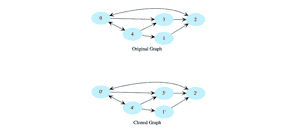Try to solve it yourself below before checking the solution.

Node* clone(Node* root) {
//TODO: Write - Your - Code
return root;
}

We use depth first traversal and create a copy of each node while traversing the graph. Use a hashtable to store each completed node so we won’t revisit nodes that exist in that hashtable. The hashtable key will be a node in the original graph, and its value will be the corresponding node in the cloned graph.

Runtime Complexity: Linear, $O(n)$

Memory Complexity: Logarithmic, $O(n)$, where $n$ is the number of vertices in the graph.

To see the solution to any of these problems in Java, Python, Ruby, or JavaScript, go to our sister-site, Coding Interview.

The goal of this exercise is to add two integers of two linked lists.

Problem statement: You are given the head pointers of two linked lists where each linked list represents an integer number (i.e. each node is a digit). Add them and return the new linked list.Try it yourself below before checking the solution.

LinkedListNode* add_integers(
//TODO: Write - Your - Code
return integer1;
}

To understand this better, let’s consider an example. Say we want to add the integers 9901 and 237. The result of this addition would be 10138.

The integers are stored inverted in the linked lists to make this easier. The most significant digit of the number is the last element of the linked list. To start adding, we start from the heads of the two linked lists.

At each iteration, we add the current digits of the two lists and insert a new node with the resulting digit at the tail of the result linked list. We’ll also need to maintain carry for each step.

We do this for all digits in both the linked lists. If one of the linked lists ends sooner, we’ll continue with the other linked list. Once both of the linked lists are done and no carry is left to be added, the algorithm will terminate.

Runtime Complexity: Linear, $O(n)$

Memory Complexity: Linear, $O(n)$

### Merge two sorted linked lists

The goal of this exercise is to merge two sorted linked lists.

Problem statement: Given two sorted linked lists, merge them so the resulting linked list is also sorted.

Try it yourself below before checking the solution.

typedef LinkedListNode* NodePtr;
//TODO: Write - Your - Code
}

Maintain a head and a tail pointer on the merged linked list. Choose the head of the merged linked list by comparing the first node of both linked lists.

For all subsequent nodes, choose the smaller current node and link it to the tail of the merged list. Move the current pointer of that list one step forward.

If there are still some elements in only one of the lists, link this remaining list to the tail of the merged list. Initially, the merged linked list is NULL.

Compare the value of the first two nodes and make the node with the smaller value the head node of the merged linked list. In this example, it is 4 from head1. Since it’s the first and only node in the merged list, it will be the tail. Then move head1 one step forward.

Runtime Complexity: Linear, $O(m + n)$, where $m$ and $n$ are lengths of our linked lists

Memory Complexity: Constant, $O(1)$

## Trees questions

### Determine if two binary trees are identical

The goal of this exercise is to compare two binary trees to determine if they are identical or not.

Problem statement: You are given the roots of two binary trees and must determine if these trees are identical. Identical trees have the same layout and data at each node.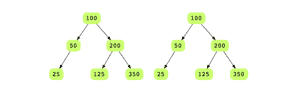Tip: Trees that have the same data aren’t necessarily identical. What’s important is their structure.

Try it yourself below before checking the solution.

bool are_identical(
BinaryTreeNode* root1,
BinaryTreeNode* root2) {
//TODO: Write - Your - Code
return false;
}

This problem can be solved recursively. The base case of recursion for this solution is if two compared nodes are null or one of them is null.

Two trees A and B are identical if:

• Data on their roots is the same or both roots are null
• The left subtree of A is identical to the left sub-tree of B
• The right subtree of A is identical to the right subtree of B

Use a depth-first traversal on both trees simultaneously and keep comparing the data at each level to solve this problem.

Runtime Complexity: Linear, $O(n)$

Memory Complexity: $O(h)$ in best case, or it will be $O(log n)$ for a balanced tree and in the worst case can be $O(n)$.

### Mirror binary tree nodes

The goal of this exercise is to use depth first traversal and bottom up mirroring to mirror the nodes of a binary tree.

Problem statement: You are given the root node of a binary tree and must swap the left and right children for each node.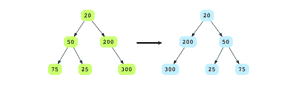Try it yourself below before checking the solution.

void mirror_tree(BinaryTreeNode* root) {
//TODO: Write - Your - Code
}

We do a post order traversal of the binary tree. For every node, swap its left child with its right child. We use DFS on the tree, so that before returning from a node, all its children have been visited (and mirrored).

Runtime complexity: Linear, $O(n)$

Memory Complexity: Linear, $O(n)$ in the worst case

To see the solution to any of these problems in Java, Python, Ruby, or JavaScript, go to our sister-site, Coding Interview.

## Strings questions

### Find all palindrome substrings

The goal of this exercise is to find the palindrome substrings of a given string.

Problem statement: Given a string, find all non-single letter substrings that are palindromes. The string given is "aabbbaa".

Try it yourself below before checking the solution.

int find_all_palindrome_substrings(string & input) {
//TODO: Write - Your - Code
return -1;
}

For each letter in the input string, start expanding to the left and right while checking for even and odd length palindromes. Move to the next letter if we know a palindrome doesn’t exist there.

We expand one character to the left and right and compare. If both are equal, we print out the palindrome substring.

Runtime complexity: Polynomial, $O(n^{2})$

Memory complexity: Constant, $O(1)$

### Reverse words in a sentence

The goal of this exercise is to reverse the words in a given string. Be sure to note how the words are separated by whitespaces.

Problem statement: Reverse the order of words in a given sentence (an array of characters). The words given are "Hello World!".

Try it yourself below before checking the solution.

void reverse_words(char * sentence) {
//TODO: Write - Your - Code
}

There are two steps to this problem. First, reverse the string. Then, traverse the string and reverse each word in place.

Runtime complexity: Linear, $O(n)$

Memory complexity: Constant, $O(1)$

Stop grinding through endless practice questions, and start breaking down real-world problems. In this course, you’ll build 100+ real product features as with hands-on coding environments inside your browser. Interview with confidence. Accelerate your career.

Decode the Coding Interview

## Dynamic programming questions

### Largest Sum Subarray

The goal of this exercise is to use your dynamic programming skills and Kadane’s algorithm to find the largest sum subarray.

Problem statement: Find the largest sum subarray. In the array below, the largest sum subarray starts at index 3 and ends at 6, and with the largest sum being 12.

Try it yourself below before checking the solution.

int find_max_sum_sub_array(int A[], int n) {
//TODO: Write - Your - Code
return -1;
}

We use Kadane’s algorithm to solve this. The basic idea of this algorithm is to scan the entire array and at each position find the maximum sum of the subarray ending there. This is achieved by keeping a current_max for the current array index and a global_max.

The algorithm is as follows:

current_max = A
global_max = A
for i = 1 -> size of A
if current_max is less than 0
then current_max = A[i]
otherwise
current_max = current_max + A[i]
if global_max is less than current_max
then global_max = current_max


Runtime complexity: Linear, $O(n)$

Memory complexity: Constant, $O(1)$

## Math and stats

### Power of a number

The goal of this exercise is to use divide and conquer and write a function that calculates the raised to the power of a number.

Problem statement: You are given a double, x and an integer n, write a function to calculate x raised to the power n.

$power (2, 5) = 32$

$power (3, 4) = 81$

$power (1.5, 3) = 3.375$

$power (2, -2) = 0.25$

Try it yourself below before checking the solution.

double power(double x, int n) {
//TODO: Write - Your - Code
return x;
}

We can use the divide and conquer approach to solve this problem most efficiently. In the dividing step, we keep dividing n by 2 recursively until we reach the base case.

In the combining step, we get the result r of the sub-problem and compute the result of the current problem using the two rules below:

• If n is even, the result is r * r (where r is the result of sub-problem)
• If n is odd, the result is x * r * r (where r is the result of sub-problem)

Runtime Complexity: Logarithmic, $O(logn)$

Memory Complexity: Logarithmic, $O(log n)$

To see the solution to any of these problems in Java, Python, Ruby, or JavaScript, go to our sister-site, Coding Interview.

### Find all sum combinations

The goal of this exercise is to use your backtracking skills to find all sum combinations.

Problem statement: Given a positive integer, target, print all possible combinations of positive integers that add to the target number.

The output will be in the form a list of lists or an array of arrays, as each element in the list will be another list containing a possible sum combination.

For example, if you are given input 5, these are the possible sum combinations.

1, 4
2, 3
1, 1, 3
1, 2, 2
1, 1, 1, 2
1, 1, 1, 1, 1


Try it yourself below before checking the solution.

vector<vector<int>> print_all_sum(int target){
vector<vector<int>> output;
return output;
}

We recursively go through all possible sum combinations, and when the running sum equals the target, print that combination. The algorithm will recursively check all the numbers that can sum up to the target.

In each recursive call, there is a for loop that runs from start to target, where start is initially 1. current_sum is the variable that is incremented with every recursive call.

Every time a value is added to the current_sum, it is also added to the result list. Whenever current_sum equals target, we know that the result list contains a possible combination for target, and this list is then appended to the final output list.

Base condition of recursion:

if current_sum equals target
print the output contents


Before each recursive call, an element is added to result. However, after each call, this element is also removed from the list to reset the list.

Runtime Complexity: Exponential, $O^2$

Memory Complexity: Linear, $O(n)$

## Searching and design questions

### Search in rotated array

The goal of this exercise is to search in a rotated array for a given number in a sorted array. Try to solve the problem using binary search.

Problem statement: Search for a given number in a sorted array, with unique elements, that has been rotated by some arbitrary number, assuming that the array does not contain duplicates. Return -1 if the number does not exist.

Below is an original array before rotation: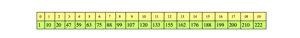After performing rotation on this array 6 times, it changes to: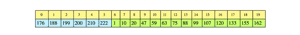Try it yourself below before checking the solution.

int binary_search_rotated(vector<int>& arr, int key) {
// TODO: Write - Your - Code
return -1;
}

The solution is like a binary search with some modifications. Notice that at least one half of the array is always sorted. If the number n lies within the sorted half of the array, then our problem is a basic binary search. Otherwise, discard the sorted half and examine the unsorted half.

Runtime complexity: Logarithmic, $O(log n)$

Memory complexity: Logarithmic, $O(log n)$

### Implement a LRU cache

The goal of this design exercise is to implement Least Recently Used (LRU), a common caching strategy, using a doubly linked list and hashing.

Problem statement: Least Recently Used (LRU) defines the policy to evict elements from the cache to make room for new elements when the cache is full, meaning it discards the least recently used items first.

Take an example of a cache that has a capacity of 4 elements. We cache the elements 1, 2, 3, and 4. This diagram below represents the cache state after first access of all four elements.

We now need to cache another element 5.

Let’s see what happens when 2 is accessed again. Now 3 becomes the next in line to be evicted from the cache.

Try it yourself below before checking the solution.

// simple single threaded LRUCache
class LRUCache {
unordered_map<int, ListNode*> cache;
// each entry in linked list is <key, value>
int capacity; // total capacity

public:

LRUCache(int capacity) {
this->capacity = capacity;
}

~LRUCache() {
for (auto& t : cache) {
delete t.second;
}
}

int get(int key) {
//TODO: Write - Your - Code
return -1;
}

void set(int key, int value) {
//TODO: Write - Your - Code
}

string print() {
string result = "";
for (auto& x : cache) {
result += "(" + std::to_string(x.first) + "," + std::to_string(x.second->value) + "),";
}
return result;
}
};

Caching is a technique to store data in a faster storage (usually RAM) to serve future requests faster. Cache stores are usually not big enough to store a full data set. We need to evict data from the cache when it becomes full.

LRU is very simple and a commonly used algorithm for this process. We evict the oldest data from the cache to accommodate new data.

To implement an LRU cache we use two data structures: a hashmap and a doubly linked list. A doubly linked list helps in maintaining the eviction order and a hashmap helps with $O(1)$ lookup of cached keys. Here is the algorithm:

If the element exists in hashmap
move the accessed element to the tail of the linked list
Otherwise,
if eviction is needed i.e. cache is already full
Remove the head element from doubly linked list and delete its hashmap entry
Add the new element at the tail of linked list and in hashmap
Get from Cache and Return



Note: The doubly linked list is for tracking the most recently accessed elements. The element at the tail of the doubly linked list is the most recently accessed element.

All newly inserted elements go to the tail, and any element accessed goes to the tail.

Runtime Complexity:

• get (hashset): Constant, $O(1)$
• set (hashset): Constant, $O(1)$
• Deletion at head when adding a new element: Constant, $O(1)$
• Search for deleting and adding to tail: Linear, $O(n)$

Memory Complexity: Linear, $O(n)$, where $n$ is the size of cache

## Behavioral questions

Now that we’ve discussed the top technical questions, let’s look at the most common behavioral interview questions you can expect at an Apple interview, which are arguably just as important for your success.

As you’ll see from this list, Apple wants to understand what kind of thinker you are, how you handle conflict, and what investments you bring to the table.

1. What were some of your best and worst days over the last four years?
2. What is your favorite Apple product or service, and why?
3. Describe an achievement you are particularly proud of.
4. Have you ever disagreed with your manager about a decision at work? What happened? How did you handle the situation?
5. How have you overcome failure? What did you learn from it?
6. Why do you want to work for Apple?
7. What is the first thing you notice when walking into an Apple store?
8. Describe the most challenging software development problem you faced. How did you solve it?
9. If you accept a job at Apple, what will you miss most about your current role? What will you miss least?
10. Do you take any steps to enhance your skills outside of work?
11. Describe a time you went above and beyond for a customer
12. Explain to a 8 year old what a modem/router is and its functions.
13. How does this role fit into your 5 year career or life plan?
14. If we hired you, what would you want to work on?
15. How would you test your favorite app?
16. If a person called for tech support but had a dinosaur or legacy product, how would you handle it?

Tip: Regardless of the question or position, it is always recommended to use STAR method for answering their behavioral-based interview questions:

• Describe the situation.
• Describe the action(s) taken to handle the task.
• Explain the results you achieved.

## Tips for preparing for interviews

Practicing for interviews takes tons of time and patience, and there is no golden ticket to cracking the coding interview. But there are some best practices we’ve learned over the years.

• Practice with different tools. It’s a good idea to combine whiteboard practice, online courses, and mock interviews to get the most out of your time. It’s crucial that you practice talking aloud as you solve problems, so use different kinds of tolls to get practice.

• Create a study plan. It is also recommended to create a detailed preparation plan for anywhere between 3-6 months. This way, you have a structure to follow and avoid missing essential concepts.

• Avoid rote memorization. It is also recommended to avoid memorizing questions. Instead, practice by building real products that Apple might use. This is the ideal way to prepare for interviews: you learn the same concepts, practice problem-solving, and gain confidence actually building for Apple.

To get hands-on practice with 100+ real product features, check out Educative’s interview prep series Decode the Coding Interview. Our team of experts has combed through the top interview questions and incorporated them into a carefully crafted set of scenarios for you to learn from.

After each project, we’ll show you what kinds of interview problems you’ll now be able to solve using the techniques you just applied.

Happy learning!